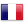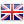﻿ Tool to convert a number from a base to another

# Tool to convert a number from a base to another

This tool changes a number from one base to another, eg decimal to binary or decimal to hexadecimal. Simply choose the base in which is the number is and the desired destination base.

The function used to perform the basic change is limited from 2 to 35. The possible characters are the numbers from 0 to 9 and the letters from a to z.
Our tool use the PHP function `base_convert`.
This function takes 3 paramters :
• Number (to be converted)
• From the base
• To the base
`base_convert` loses accuracy on large numbers and decimal numbers. Indeed, numbers are converted into binary to be computed and these representations have a limited length. Not all numbers can be represented accuratly by a computer. This concerns especially the decimals.FREN